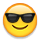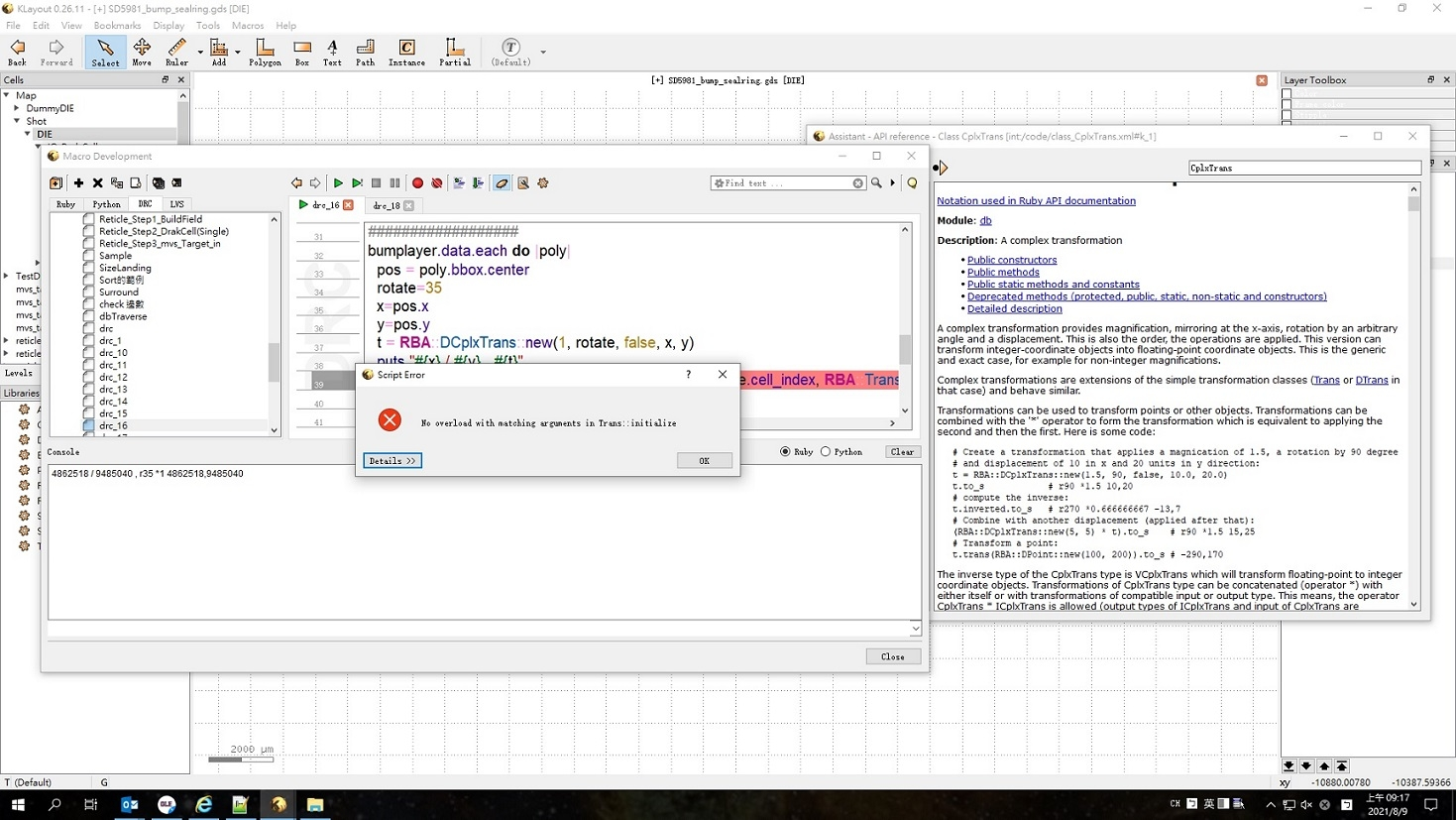# How to Make a instance in DRC script?

as the below DRC script , I can create a cell "Map"
can we do more script to make some cell instance into this cell?
such as
`out_cell.insert(RBA::DCellInstArray::new(subcell_index, RBA::DTrans::new(locationx , locationy)))`

``````layout = RBA::Application::instance.main_window.current_view.active_cellview.layout
top = layout.create_cell("Map")
l1 = layout.layer(1, 0)
dbu = layout.dbu.round(4)
map = 150000.00
polygon = 2000
ebrsize= map - 3000.00

mapsize = map / dbu
mapcenter = mapsize / 2.00
top.shapes(l1).insert(RBA::Box::new( -mapcenter , -mapcenter , mapcenter  , mapcenter ))
``````

• edited July 2021

This isn't a DRC script - it's a plain API script. Run that as "Ruby Macro".

And yes, within such a script, you can generate cells and instances.

Matthias

• Hi Matthias,
how to do DRC in Ruby scribe?
here is my sample code ,
but I can't do that in Ruby scribe ..
in fact , I want to using DRC function to get some pattern location / then do some caculate and make instance base on the result.
but look like that is not-work in Ruby (that is workable in DRC , but didn't work in Ruby...)

``````################################################
include RBA
main_window = RBA::Application::instance.main_window
layout = RBA::CellView::active.layout
layout_view = main_window.current_view
layout = layout_view.active_cellview.layout
layer = layout.layer(86, 0)
layer.each do |X|
ubmX1=X.bbox.p1.x
ubmY1=X.bbox.p1.y
puts "I am get answer #{ubmX1} and #{ubmY1}"
end
``````
• Hi @jiunnweiyeh,

here is some sample code how to do cell placement in DRC. It places a cell called "PLACEME" over all locations where layer 45/0 overlaps with layer 37/0:

``````placeme = source.layout.cell("PLACEME")

layer1 = input(45, 0)
layer2 = input(37, 0)

(layer1 & layer2).data.each do |poly|

pos = poly.bbox.center

source.cell_obj.insert(RBA::CellInstArray::new(placeme.cell_index, RBA::Trans::new(pos)))

end
``````

You should place the cell instance generation at the end of the script - I'm not sure how the new cells will interact with input taken after placing them.

Matthias

• Hi Matthias,
Thanks for your code , I will check it .

• Hi Matthias,
The code is workable , but how about make a cell array (2x2 , row vator/ col vator as 5000,5000)
and make the cell instance rotate by 90 degree ?

• @jiunnweiyeh

That's

``````RBA::CellInstArray::new(0, RBA::Trans::new(1, false, RBA::Vector::new(0, 0)), RBA::Vector::new(0, 1000), RBA::Vector::new(1000, 0), 2, 2)
``````

Matthias

• Hi Matthias,
It is workable and thanks very much for your help.

• Hi Matthias sir,
Another question , if I want to ....
1.base on current active cell , do some layer boolean , such as xor ...not...,
then move the result pattern to a new cell , how can I do that ?

2.in current layer map , I have a layer name as 1/0 (I have some pattern in this layer in other cell (cell) .
how to do layer boolean in current cell (cell A) , but keep layer 1/0 pattenr in cell B?

• @jiunnweiyeh

In general, DRC is not optimized for cell specific operations. The concept of DRC is that of layers stretching across all cells and the hierarchy. This abstraction is crucial.

You can use the "Region" class for doing what you want, but that's no longer DRC. If you want detailed operations, you need to stop using DRC and start writing Pyton or Ruby scripts.

Matthias

• Hi Matthias,
Thanks , got it.
By the way , cause I want to make the instance by a special degree , such as 35.degree.
but I can't do that as this sample.
how can I do to make that ?

``````      rotate=R35
source.cell_obj.insert(RBA::CellInstArray::new(placeme.cell_index, RBA::Trans::new(rotate, true, RBA::Vector::new(pos))))
``````
• @jiunnweiyeh That is a complex transformation instead of a RBA::Trans (RBA::CplxTrans). This allows using any angle and magnifications too.

• Hi Matthias,
sorry , could you please help to provide a single sample for how to using that function ?
I try to using the sample code in "help" , that is as below...
But I have no idea how to using the value "t" in the insert cell function.

``````    layout = RBA::CellView::active.layout
placeme = source.layout.cell("CapRD")
arraycell="UU_array"
removebumplayer = "No"
bumplayer.data.each do |poly|
pos = poly.bbox.center
rotate=35
x=pos.x
y=pos.y
t = RBA::DCplxTrans::new(1, rotate, false, x, y)
puts "#{x} / #{y} , #{t}"
source.cell_obj.insert(RBA::CellInstArray::new(placeme.cell_index, RBA::Trans::new(t, true, RBA::Vector::new(pos))))
end
puts "Finished Job"
``````• Replace

``````RBA::Trans::new(...)
``````

by the corresponding flavor of RBA::CplxTrans (RBA::ICplxTrans):

``````# mag: scaling factor (1.0 usually)
# angle: rotation angle in degree(!)
# mirror, dx, dy: as in RBA::Trans
RBA::ICplxTrans::new(mag, angle, mirror, RBA::Vector::new(dx, dy))
``````

Matthias

• Hi Matthias,
I want to do taht...
1.base on the xxx layer pattern(bbox), to make cell instance into current view.
2.change (replace) the cell instace rotate degree.
so , that is what my code .... I want to make 1 request in same time....but the code is fail...

``````    bumplayer.data.each do |poly|
pos = poly.bbox.center
rotate=35
dx=pos.x
dy=pos.y
mag=1.0
angle=10
mirror="true"
source.cell_obj.insert(RBA::ICplxTrans::new(mag, angle, mirror, RBA::Vector::new(dx, dy)))
end
``````
• First, you should use "true" (without quotes) for mirror:

``````mirror = true
``````

Second, you still need to generate a CellInstArray. Just substitute "RBA::Trans" by "RBA::ICplxTrans", not the whole CellInstArray call like

``````      source.cell_obj.insert(RBA::CellInstArray::new(placeme.cell_index, RBA::ICplxTrans::new(...)))
``````

Matthias

• Hi Matthias,
Thanks a lot , it is workable.